Courses

# Logarithms CA Foundation Notes | EduRev

## CA Foundation : Logarithms CA Foundation Notes | EduRev

The document Logarithms CA Foundation Notes | EduRev is a part of the CA Foundation Course Quantitative Aptitude for CA CPT.
All you need of CA Foundation at this link: CA Foundation

Logarithm

The logarithm of a number to a given base is the index or the power to which the base must be raised to obtain that number.

If am  = b (where b > 0, a ≠ 1, a > 0), then the exponent m is said to be logarithm of b to the base a.

So, if am = b       (Exponent form)

Then, m = loga b   (Logarithm form)

Example 1. Express in logarithmic form: (i) 23  (ii) xm = y

Solution : 23 = 8 ⇒ log28 = 3

xm = y ⇒ logxy = m

Note:

• Logarithm of any quantity to the same base is 1.
• Logarithm of 1 to any base is zero

Types of Logarithm

1. Logarithms to the base “e” (exponential constant) are called natural logarithms.

2. Logarithms to the base “10” are called common logarithms

Note: If the base is not given, it can be assumed to be 10

Laws of Logarithm

1. loga(mn) = loga m + loga n

Logarithm of a product of two numbers can be written as sum of logarithms of the individual numbers.

Example 1.  If log10 2 = 0.3010, log10 3 = 0.4771, find log10 6.

Solution : log10 6 =  log10 (2x3)

log10 2=  log10 3

= 0.3010 + 0.4771 = 0.7781

Example 2. Simplify log 2 + log 3 + log 4

Solution : log 2 + log 3 + log 4 = log (2 × 3 × 4) = log 24

2.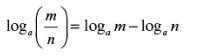Logarithm of a division of two numbers can be written as difference of logarithms of the individual numbers.

Example: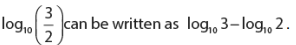3. (i) logamn = n logam

(ii)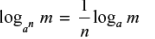Logarithm of the nth  power of a number is n times the logarithm of that number.

Logarithm of a number m to the base a raised to power n is equal to 1/n times the logarithm of m to the base a.

Example 1 :  If log10 2 = 0.3010, find log10 8.

Solution : log108 = log102 = 3 log10 2 = 3 × 0.3010 = 0.9030

Example 2.  Find the value of 1/2log10 = 100

Solution :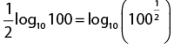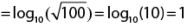4.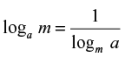Example :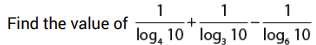Solution :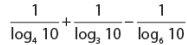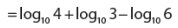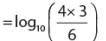= log10(2)

5.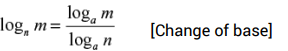We can write any logarithm as a division of two logarithms (of the number and the base) taken at any common base. This theorem is very useful in solving problems having logarithms with many different bases.If we put m = a in the above result, we get another important result which is logn a × loga n = 1

Example : Find the value of log3 7 x log49 243

Solution :  To simplify, we will make use of change of base theorem and convert each term into a logarithm with common base of 10.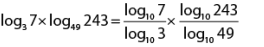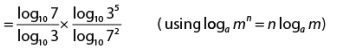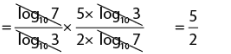6. If loga m = x, then

a. log(1/a)  m = -x

b.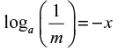c.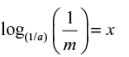Offer running on EduRev: Apply code STAYHOME200 to get INR 200 off on our premium plan EduRev Infinity!

66 docs|68 tests

,

,

,

,

,

,

,

,

,

,

,

,

,

,

,

,

,

,

,

,

,

;